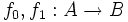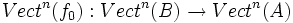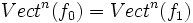# Vector bundle class functor is homotopy-invariant for paracompact

This article describes a result applicable for real vector bundles over a paracompact Hausdorff space. In particular, the result is applicable for real vector bundles over a manifold, CW-space, or metrizable space

## Statement

Suppose$A$ and$B$ are paracompact Hausdorff spaces and$f_0, f_1: A \to B$ are continuous maps. Then, the functorially induced maps by the vector bundle class functor, namely:$Vect^n(f_0): Vect^n(B) \to Vect^n(A)$

and:$Vect^n(f_1): Vect^n(B) \to Vect^n(A)$

are equal, i.e.$Vect^n(f_0) = Vect^n(f_1)$.

## Corollaries

• Any homotopy equivalence of paracompact Hausdorff spaces induces an isomorphism on the vector bundle classes over them.
• In particular, any vector bundle over a contractible paracompact Hausdorff space is trivial.